Author: Oscar Cronquist Article last updated on May 06, 2019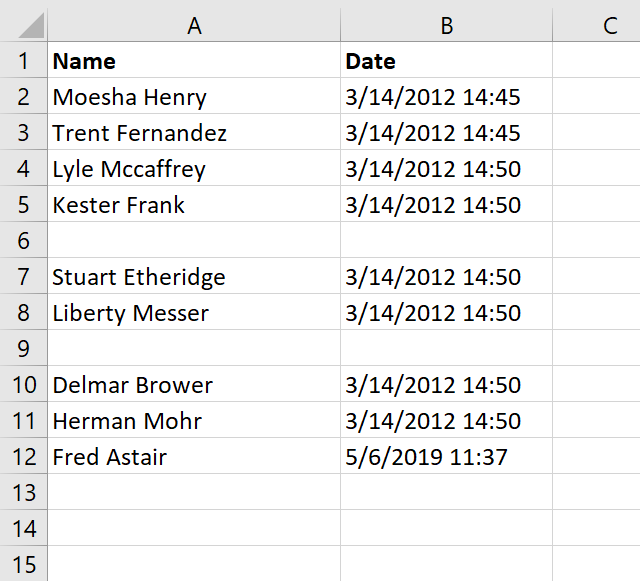This article demonstrates how to automatically enter data in cells if an adjacent cell is populated using VBA code.

In some cases, it can be useful and timesaving to automate data entering, the VBA examples here all enter a value or formula in a cell if a value is entered by the user in the adjacent column.

The image above shows a worksheet that enters a timestamp in column B if the corresponding cell in column A is populated.

### VBA enters timestamp automatically in the adjacent cell

Enter a name in column A and current date and time is entered automatically in column B. You can also copy a cell range and paste them to column A. Empty cells are not processed.

What makes this work is an event code that catches changes on a worksheet, this makes it possible to populate the adjacent cell with whatever you want. I am in this case entering a time stamp in column B.

#### VBA code

```'Event procedure that is executed if any cell on the worksheet is changed
Private Sub Worksheet_Change(ByVal Target As Range)

'Dimension variable and data type
Dim Value As Variant

'If target cell (the cell that changed) is in column A then do this.
If Not Intersect(Target, Range("A:A")) Is Nothing Then

'Repeat these lines as many times as there were cells that changed
For Each Value In Target

'If value is not equalt to nothing then do this.
If Value <> "" Then

'Populate adjacent cell in column B based on row number from target cell
Range("B" & Value.Row).Value = Now
End If
Next Value
End If
End Sub
```

#### Where to copy code?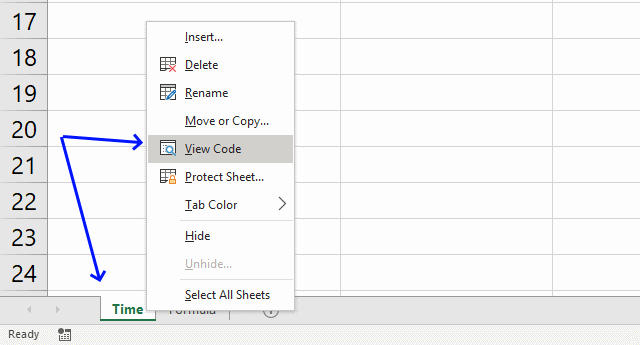1. Right click on the sheet name
2. Click "View code" to open the VB Editor.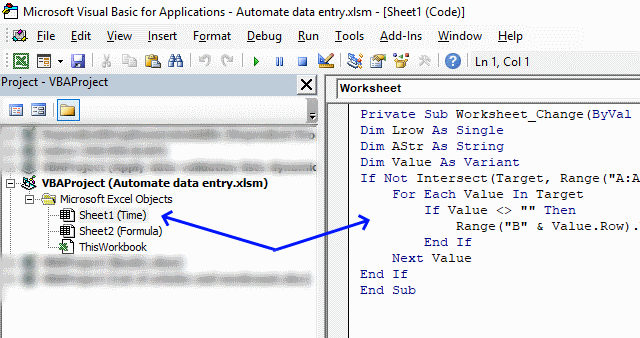3. Copy/Paste vba code to sheet module, see image above.

### Populate a cell with a formula using VBA

The following VBA code populates the adjacent cell in column C with a formula if corresponding cell value in column B is populated.

Here is what I did: Enter a price in column B and a formula is instantly entered in column C. Formula in column c: Cell value in column B multiplied by 1.1

#### VBA code

```'Event code that runs if a cell or cell range is changed
Private Sub Worksheet_Change(ByVal Target As Range)

'Dimension variables and declare data types
Dim Lrow As Single
Dim AStr As String
Dim Value As Variant

'Check if cell or cell range that changed is in column B
If Not Intersect(Target, Range("B:B")) Is Nothing Then

'Repeat the following code as many times as there a cells in the cell range that changed
For Each Value In Target

'Check if cell (that changed) is not equal to nothing
If Value <> "" Then

'Populate adjacent cell in column C with a formula
Range("C" & Value.Row).Formula = "=" & Target.Address & "*1.1"
End If
Next Value
End If
End Sub
```

#### Where to copy code?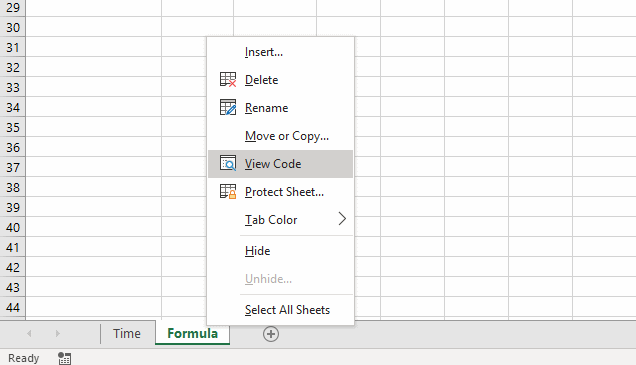1. Right click on current sheet name
2. Click "View code"
3. Copy/Paste vba code to sheet module.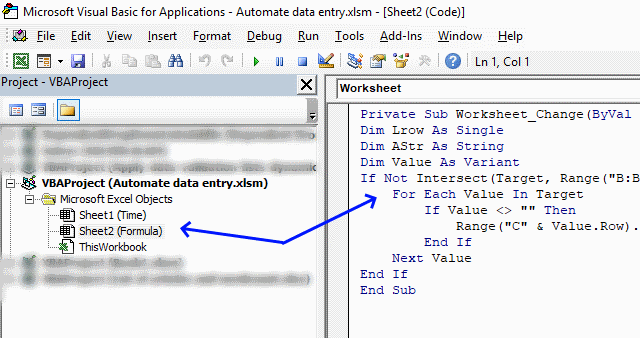4. Copy/Paste vba code to sheet module, see image above.

### Final notes

The techniques demonstrated here in this article should give you a great start in automating data entry in your workbook.

Keep in mind that if you happen to get caught in an endless loop because the code changes a value that then triggers the event code over and over you can prevent this by using the Application.EnableEvents property.

```Application.EnableEvents = False

Code

Application.EnableEvents = True

```

This will stop the event code from triggering while the macro manipulates the worksheet.

The first example above automatically enters the timestamp, however, if want to know how to manually enter a timestamp then use following shortcut keys: CTRL + :

The second example can be done using formulas as well, you can also use a formula that will output nothing if the adjacent value is empty.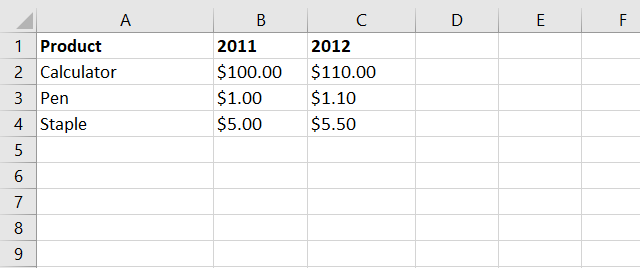Formula in cell C2:

=IF(B2<>"",B2*1.1,"")

This formula will check if cell B2 is not empty and then multiply that value with 1.1 if TRUE and return a blank if FALSE.

B2<>"" is a logical expression that returns either TRUE or FALSE. If B2 is not empty the logical expression returns TRUE and if it is empty the logical expression returns FALSE.

IF(logical_expression, if_true, if_false)

The IF function then runs the second argument if the logical expression returns TRUE and the third argument if FALSE.

You can read more about this function here: IF function

### Download Excel file

* You will also get a weekly newsletter, unsubscribe anytime!